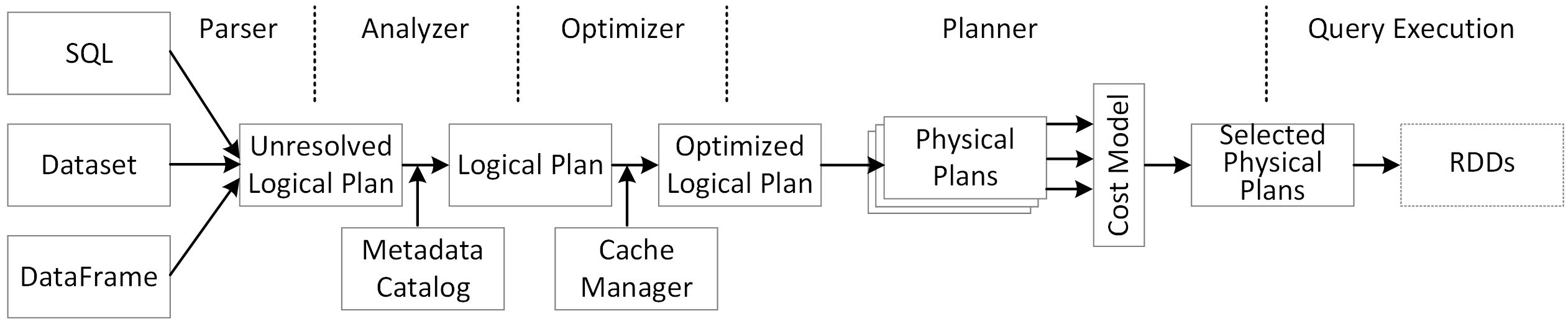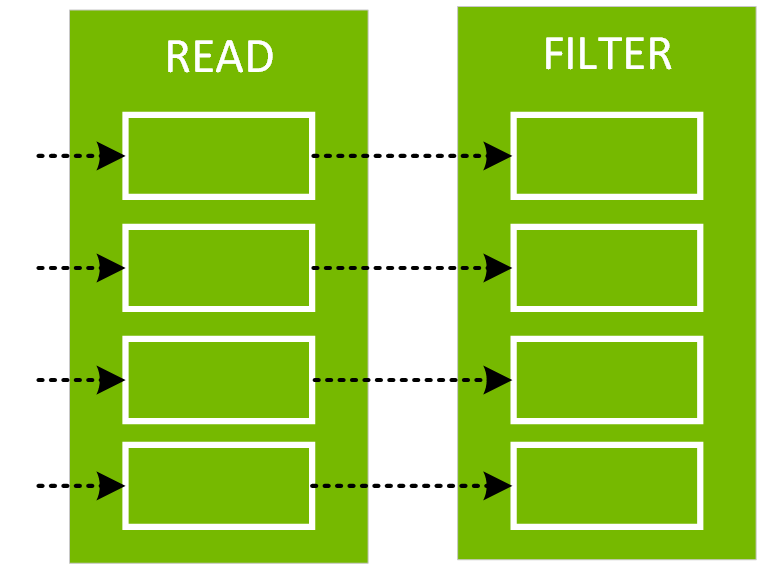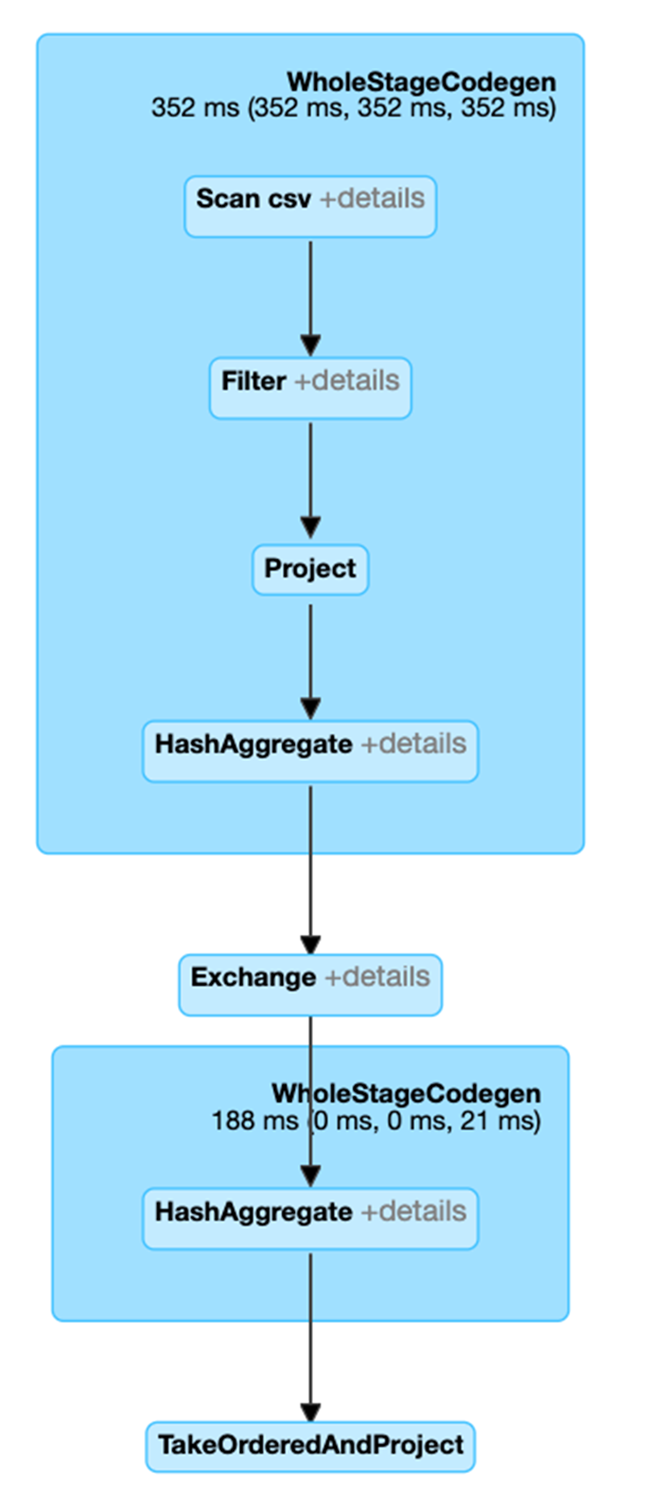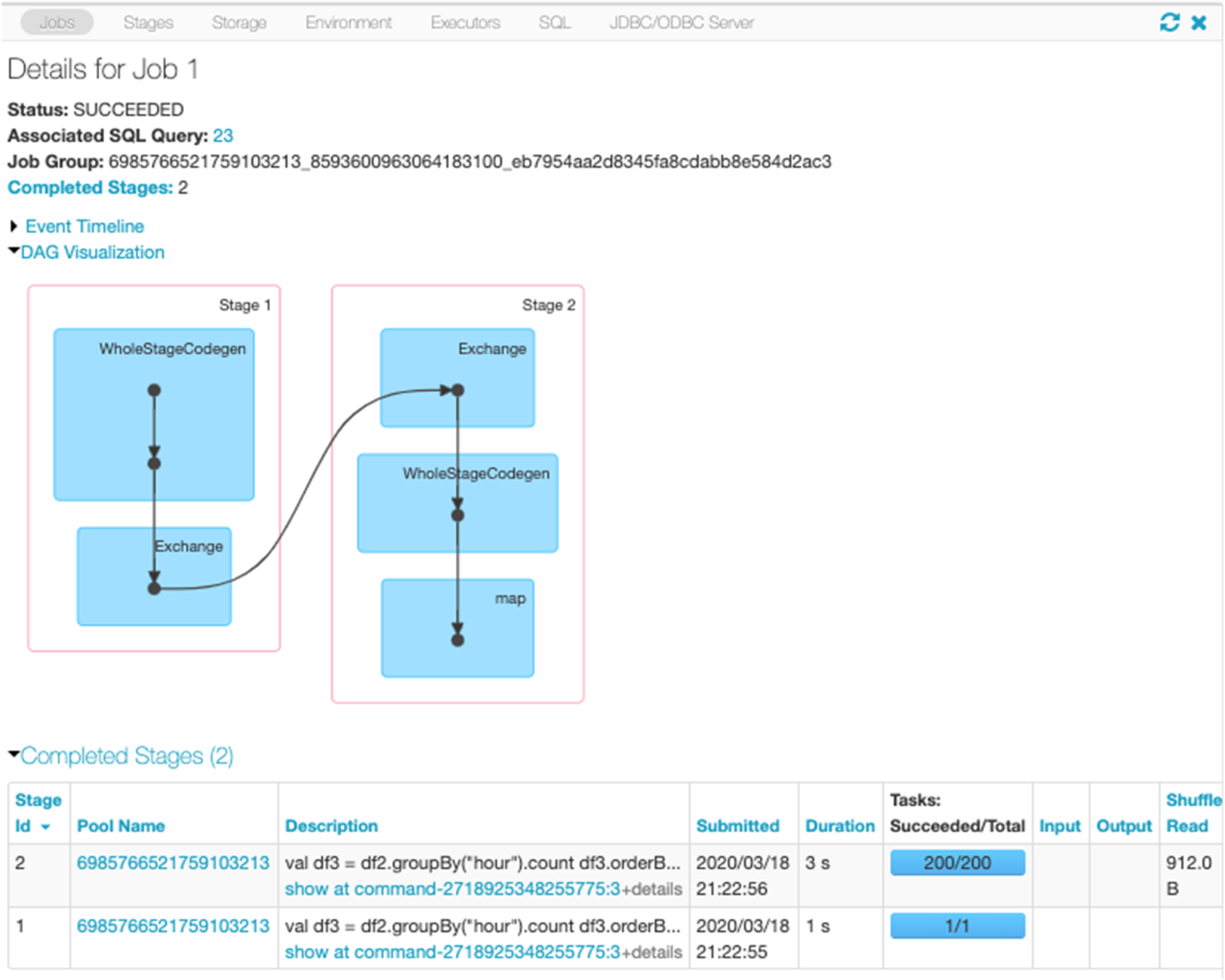### Spark 應用程式如何執行？

• 建立邏輯計畫
• 將邏輯計畫轉換為實體計畫
• 產生程式碼
• 在叢集上執行工作### 查看實體計畫

val df2 =df.select(\$"hour", \$"fare_amount", \$"day_of_week").filter(\$"day_of_week" === "6.0" )
df2.show(3)
result:
+----+-----------+-----------+
|hour|fare_amount|day_of_week|
+----+-----------+-----------+
|10.0|       11.5|        6.0|
|10.0|        5.5|        6.0|
|10.0|       13.0|        6.0|
+----+-----------+-----------+
df2.explain(“formatted”)
result:
== Physical Plan ==
* Project (3)
+- * Filter (2)
+- Scan csv  (1)

(1) Scan csv
Location: [dbfs:/FileStore/tables/taxi_tsmall.csv]
Output : [fare_amount#143, hour#144, day_of_week#148]
PushedFilters: [IsNotNull(day_of_week), EqualTo(day_of_week,6.0)]

(2) Filter [codegen id : 1]
Input : [fare_amount#143, hour#144, day_of_week#148]
Condition : (isnotnull(day_of_week#148) AND (day_of_week#148 =6.0))

(3) Project [codegen id : 1]
Output : [hour#144, fare_amount#143, day_of_week#148]
Input : [fare_amount#143, hour#144, day_of_week#148]val df3 =df2.groupBy("hour").count
df3.orderBy(asc("hour"))show(5)
result:
+----+-----+
|hour|count|
+----+-----+
| 0.0|   12|
| 1.0|   47|
| 2.0|  658|
| 3.0|  742|
| 4.0|  812|
+----+-----+

df3.explain
result:
== Physical Plan ==
* HashAggregate (6)
+- Exchange (5)
+- * HashAggregate (4)
+- * Project (3)
+- * Filter (2)
+- Scan csv  (1)
(1) Scan csv
Output : [hour, day_of_week]
(2) Filter [codegen id : 1]
Input : [hour, day_of_week]
Condition : (isnotnull(day_of_week) AND (day_of_week =6.0))
(3) Project [codegen id : 1]
Output : [hour]
Input : [hour, day_of_week]
(4) HashAggregate [codegen id : 1]
Input : [hour]
Functions : [partial_count(1) AS count]
Aggregate Attributes : [count]
Results : [hour, count]
(5) Exchange
Input : [hour, count]
Arguments: hashpartitioning(hour, 200), true, [id=]
(6) HashAggregate [codegen id : 2]
Input : [hour, count]
Keys : [hour]
Functions : [finalmerge_count(merge count) AS count(1)]
Aggregate Attributes : [count(1)]
Results : [hour, count(1) AS count]### 在叢集上執行工作